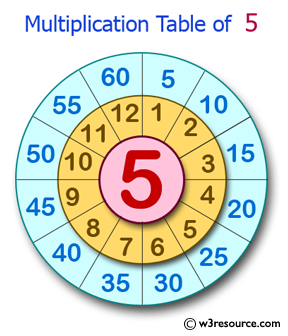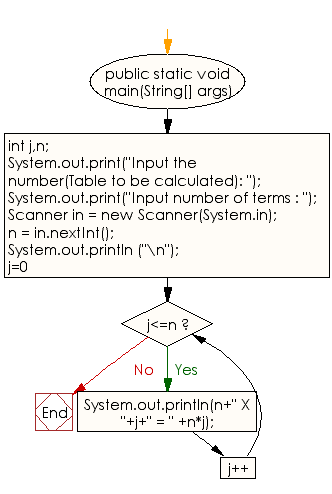﻿ Java exercises: Display the multiplication table of a given integer - w3resource# Java Conditional Statement Exercises: Display the multiplication table of a given integer

## Java Conditional Statement: Exercise-14 with Solution

Write a program in Java to display the multiplication table of a given integer.

Test Data
Input the number (Table to be calculated) : Input number of terms :5

Pictorial Presentation:Sample Solution:

Java Code:

``````import java.util.Scanner;
public class Exercise14 {

public static void main(String[] args)

{
int j,n;

System.out.print("Input the number(Table to be calculated): ");
{
System.out.print("Input number of terms : ");
Scanner in = new Scanner(System.in);
n = in.nextInt();

System.out.println ("\n");
for(j=0;j<=n;j++)

System.out.println(n+" X "+j+" = " +n*j);
}
}
}
```
```

Sample Output:

```Input the number(Table to be calculated): Input number of terms : 5
5 X 0 = 0
5 X 1 = 5
5 X 2 = 10
5 X 3 = 15
5 X 4 = 20
5 X 5 = 25
```

Flowchart:Java Code Editor: